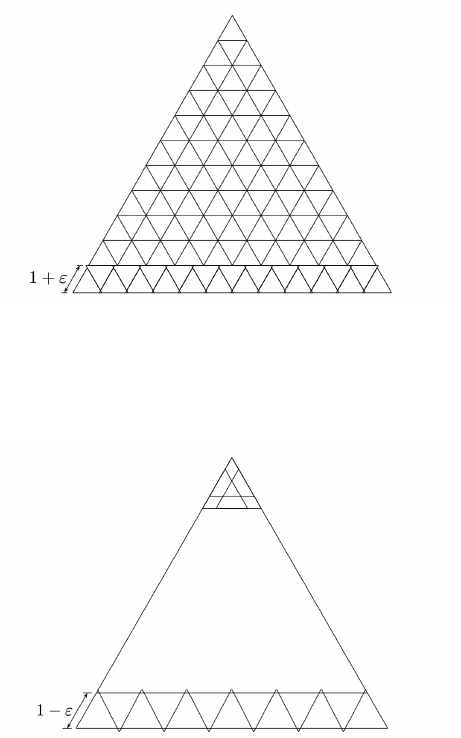This paper is the shortest paper ever submitted to a serious journa...
Let us first try to determine using area considerations what is the...
This is the way John Conway found to prove that you need $n^2+2$ un...
This is the construction that Soifer came up with to prove the same...Can n
2
+ 1 unit equilateral triangles
cover an equilateral triangle of side > n,
say n + ε?
John H. Conway , Alexander Soifer
Princeton University, Mathematics, Fine Hall, Princeton, NJ 08544, US
n
2
+ 2 can:
Figure 1:
Figure 2:
1
I get the proofs, but what's the point of this paper though? Is it just there because the proofs were a kind of fun? Or does it have deeper applications in some mathematical fields? Let us first try to determine using area considerations what is the minimum number of unit equilateral triangles we must have. $$A_{Small \ \triangle}=\frac{\sqrt3}{4} \\ A_{Big \ \triangle}=\frac{\sqrt3}{4}(n+\varepsilon)^ 2= A_{Small \ \triangle}(n^2+2n\varepsilon+\varepsilon^2) \\ <A_{Small \ \triangle}(n^2+1)$$ We conclude that we need at least $n^2+1$ unit triangles just using area considerations (assuming $\varepsilon$ is sufficiently small). Are you suggesting that the authors prove that $n^2+2$ triangles are needed? I don't read the article as claiming that: the authors show that $n^2+2$ are enough, and clearly (as by the area considerations you wrote above) at least $n^2+1$ are needed, but they don't show that other arrangements of $n^2+1$ small triangles don't cover the big triangle. That's the reason that the title of the article is a question. This paper is the shortest paper ever submitted to a serious journal. The paper has only 2 words and 2 drawings and was written by John Conway and Alexander Soifer in 2004. ![Conway and Soifer](http://media.bastillepost.com/wp-content/uploads/hongkong/2015/04/20150422-PN_%E5%AD%B8%E8%A1%93%E8%AB%96%E6%96%87_%E6%9C%80%E7%9F%AD-5a.jpg) The idea for the paper came during one of the famous coffee hours at Princeton's math department. This is the way John Conway found to prove that you need $n^2+2$ unit equilateral triangles to cover the big triangle. To understand his proof let us analyze the simpler case: $n=3$. In this situation, you can start stacking unit triangles from the top and you will end up with something like this: ![](http://i.imgur.com/kDab6bV.png) We can see that we still need to cover up a trapezoid where the size of the outer sides is $1+\varepsilon$. The way to cover this trapezoid is by stacking 2 slightly misaligned unit triangles on top of each other so that we can cover the extra space "caused" by $\varepsilon$ and then repeat the process until we cover the entire trapezoid. As can be seen in the figure, the fact that the trapezoid has the sides enlarged by a factor of $\varepsilon$ forces us to use 2 additional triangles to cover it completely. ![](http://i.imgur.com/UJHvEIY.png) ![](http://i.imgur.com/8nICP17.png) If $\varepsilon=0$, as we have demonstrated in the area consideration above, we need exactly $3^2$ unit triangles to cover the big triangle and when $\varepsilon\neq 0$ we need 2 extra triangles so: $3^2+2$. It's not difficult to see that this construction can be generalized for a triangle of arbitrary size: $n+\varepsilon$ and so the total number of unit triangles is $n^2+2$. This is the construction that Soifer came up with to prove the same result. To understand it we will use again a simpler case and then generalize the conclusions. Let us first start with a triangle with sides $2+\varepsilon$. To cover this triangle we start by using 3 unit triangles to place in the corners. As can be seen in the figure we are left with an hexagon in the middle.To cover the remaining hexagon we need 3 unit triangles arranged in the following way: ![](http://i.imgur.com/yrb3zcx.png) For $n=2$, Soifer's construction is as "efficient" as Conway's, since we ended up using $2^2+2$ unit triangles to cover a triangle of side $2+\varepsilon$. Now for $n=3$, we will do exactly the same thing we did for $n=2$, but the space in the "middle" will be $2\varepsilon$. As a consequence we are left with a trapezoid where the size of the outer sides is $1-\varepsilon$. figure To cover the trapezoid we use the same technique used by Conway but this time we don't need any extra unit triangles to cover it. Note that this is only possible because the outer side is $1-\varepsilon$, otherwise we would have needed more triangles to cover the trapezoid, since the length of the base is $3+\varepsilon$ (that's why we left $2\varepsilon$ in the middle). ![](http://i.imgur.com/Kk0OjmK.png) The total number of unit triangles we used to cover the $n=3$ case was again $3^2+2$, which is equivalent to Conway's construction. It's not hard to see that this result can be generalized for an arbitrary $n$, and so the total number of unit triangles is $n^2 + 2$. We just need to use the 3 triangle structure and make sure the trapezoid at the bottom has a size $1-\varepsilon$ (Soifer chose to start with the 3 triangle structure as can be seen in Figure 2). The point of this paper is to ask the question in the title of the paper; that is why it gets away with such a short word-count. If this were a typical-format paper, it would probably state: "It is well known that $n^2$ unit equilateral triangles will perfectly tile the equilateral triangle of size $n$, and this fact also comes from a simple scaling law: if the area of a unit triangle is $\alpha$ then simple scaling by a factor of $n$ gives a total area of $n^2\alpha$ requiring $n^2$ unit triangles. We present two constructions that $n^2 + 2$ triangles are sufficient, leaving an open question: whether there is any $\epsilon$ such that $n^2 + 1$ triangles tile a triangle of side length $n^2 + \epsilon$."